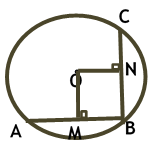Maths / Circles / Equal Chords are Equidistant from the Center of Circle

QUESTION

 In the given, O is the centre of the circle, AB = BC = 4 units and ON = 4.5 units. The value of OM + MB is equal toOPTIONS A. 4.5 units B. 6.5 units C. 2 units D. 4.7 units
Right Option : B

EXPLANATION
Explain TypeExplanation Content
Text

As equal chords are equidistant from the centre and AB = BC and the distancde of BC i.e. ON= 4.5 units

so AB is also at a distance of 4.5 units

OM= 4.5 units

The perpendicular bisects the chord as AB = 4units so MB = 2units

OM + MB = 4.5+2 = 6.5 units

TestimonialsSTUDENT FEEDBACK - Aman Kumar Shrivastava C/o ABHYAS Academy
10th
The experience was nice. I studied here for three years and saw a tremendous change in myself. I started liking subjects like English and SST which earlier I ran from. Extra knowledge gave me confidence to overcome competitive exams. One of the best institutes for secondary education.